Question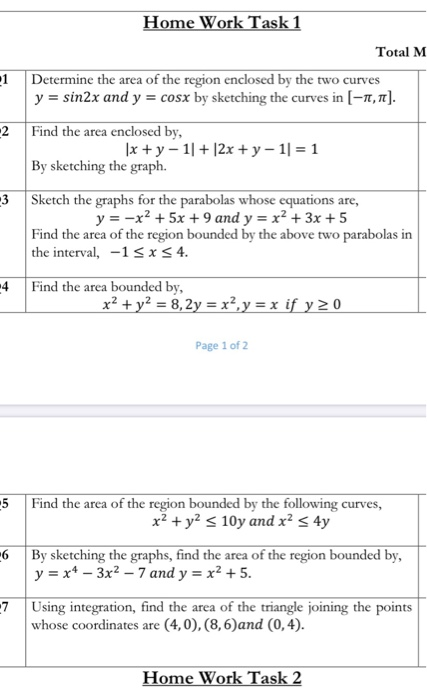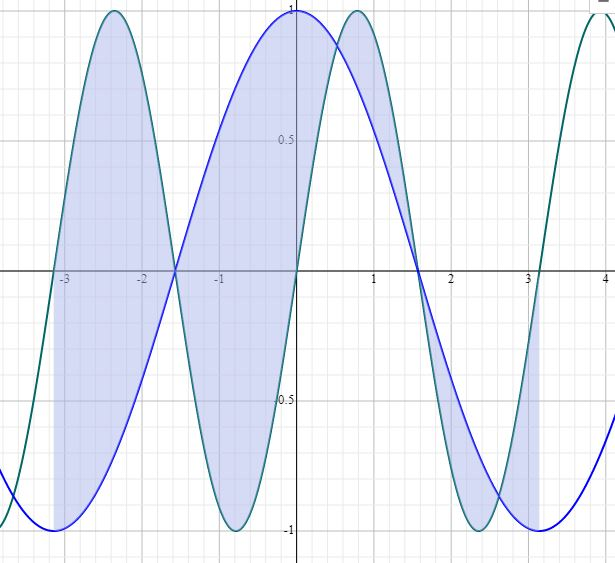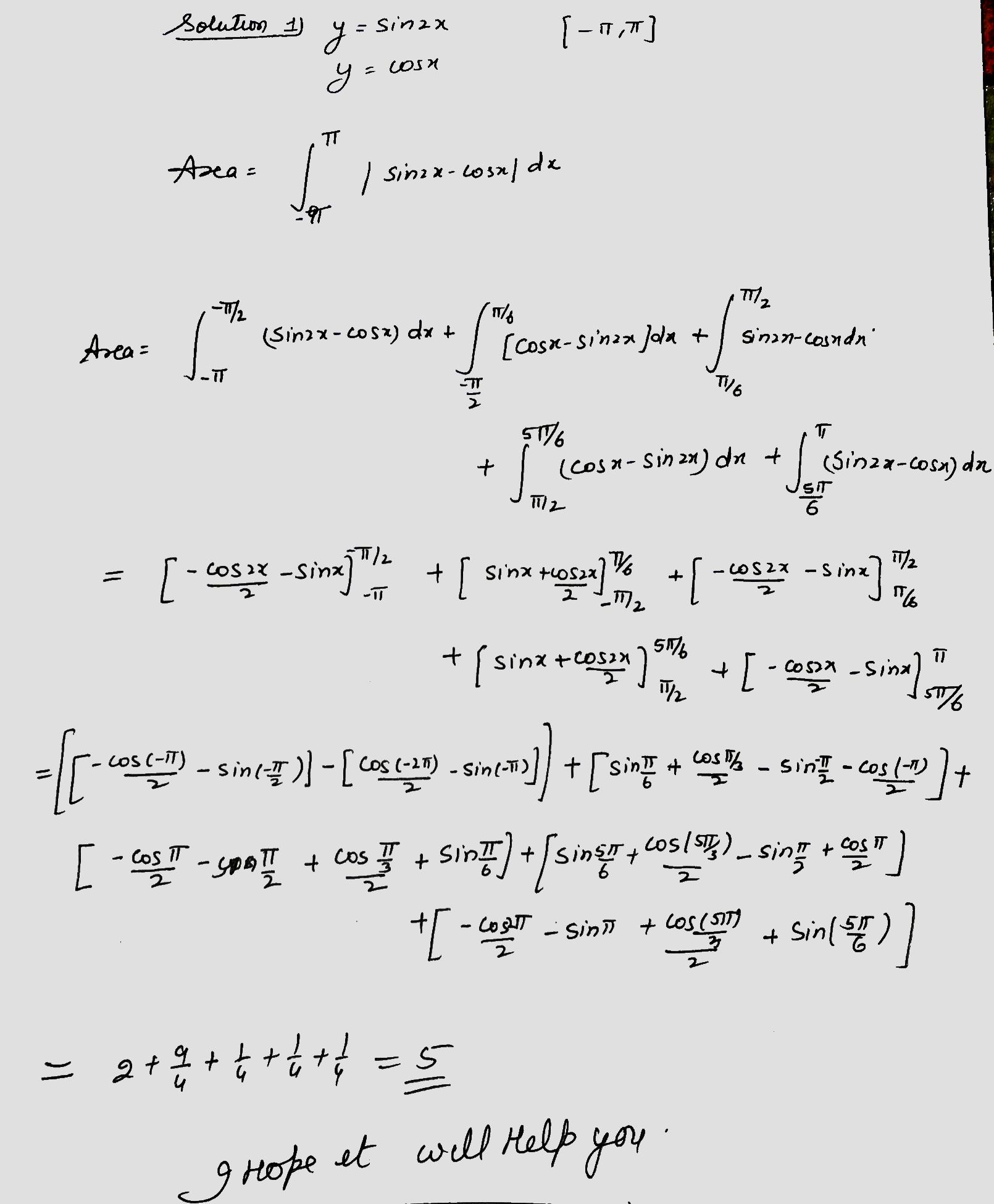#### Earn Coins

Coins can be redeemed for fabulous gifts.

Similar Homework Help Questions
• ### plzzz Q6 By sketching the graphs, find the area of the region bounded by, y =...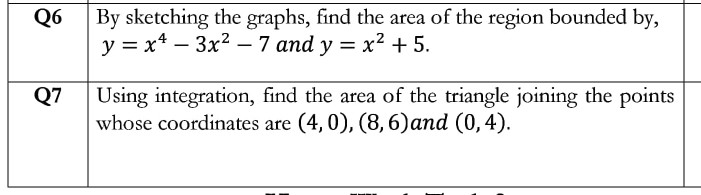plzzz Q6 By sketching the graphs, find the area of the region bounded by, y = x4 - 3x2 – 7 and y = x2 + 5. 07 Using integration, find the area of the triangle joining the points whose coordinates are (4,0), (8,6)and (0,4).

• ### show all work 1. Find the area of the region bounded by the curves below. Sketch...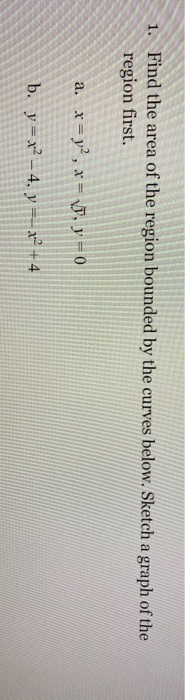show all work 1. Find the area of the region bounded by the curves below. Sketch a graph of the region first. a. x = y2, x = VD, y = 0 b. y = x2 – 4, y == x2 + 4

• ### #2 g and #3&4 please!!! #2 For each of the following, first sketch (roughly) the region...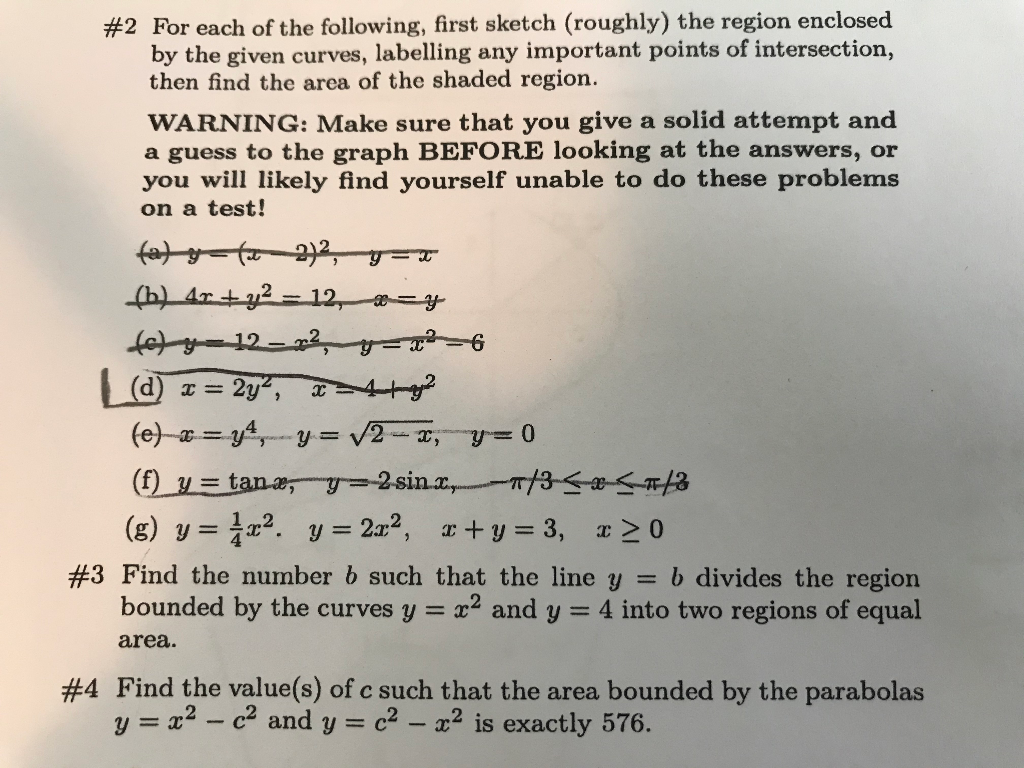#2 g and #3&4 please!!! #2 For each of the following, first sketch (roughly) the region enclosed by the given curves, labelling any important points of intersection, then find the area of the shaded region. WARNING: Make sure that you give a solid attempt and a guess to the graph BEFORE looking at the answers, or you will likely find yourself unable to do these problems on a test! (a)--(-2)2,--- (h) 4x + y2 = 12, *= fety-12-22 - x²6...

• ### 16 pts) 1. Determine the area of the region between the two curves y=x and y+2x...16 pts) 1. Determine the area of the region between the two curves y=x and y+2x by integrating over the x-axis. Hint: Refer the figure and note that you will have two integrals to solve by splitting the region between the two curves into two smaller regions. lo pl [6 pts) 2. Find the area of the region bounded by the curves y=12 - x, y=vx, and y20

• ### Problem 2 (1) Find the area enclosed by the curves y 2 and y-4z-z2 (2) Find the volume of the solid whose base is the triangular region with vertices(0, 0), (2, 0), and (0,1). Cross-sections perpendi...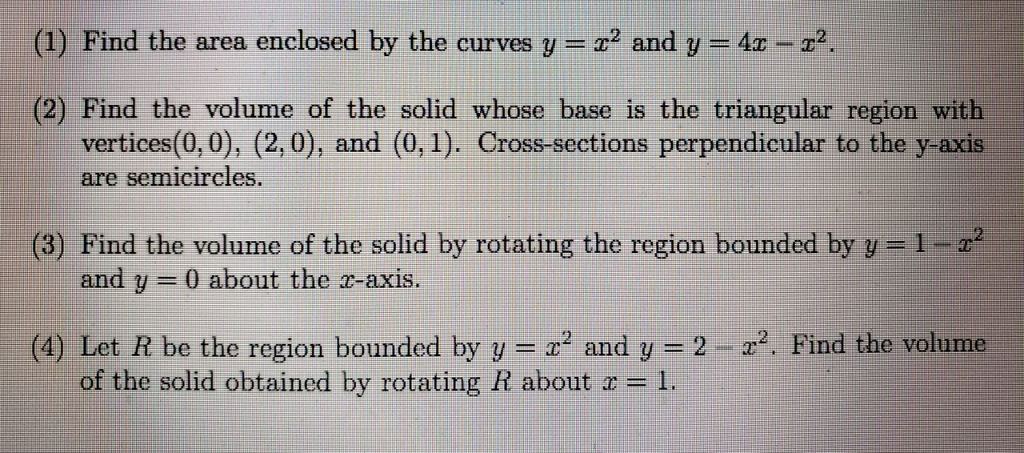Problem 2 (1) Find the area enclosed by the curves y 2 and y-4z-z2 (2) Find the volume of the solid whose base is the triangular region with vertices(0, 0), (2, 0), and (0,1). Cross-sections perpendicular to the y-axis semicircles. are (3) Find the volume of the solid by rotating the region bounded by y=1-z2 and y-0 about the r-axis. 2-z2. Find the volume (4) Let R be the region bounded by y--x2 and y of the solid obtained by...

• ### 2. (a) Find the area of the region bounded by the parabolas y 6z - 2 and y 22 (b) (Optional) Find...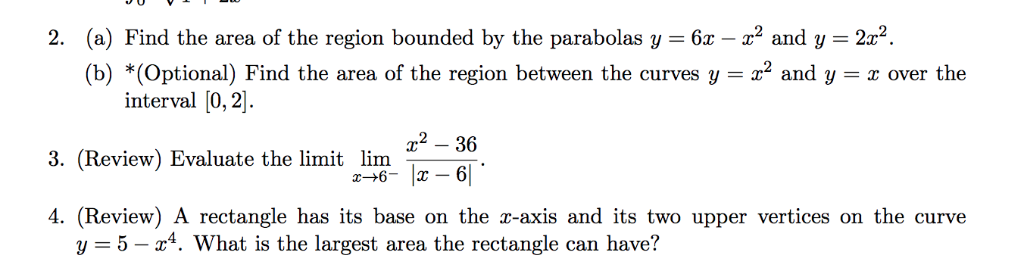2. (a) Find the area of the region bounded by the parabolas y 6z - 2 and y 22 (b) (Optional) Find the area of the region between the curves y2 and y - 22".. over the interval [0,2. 2 36 3. (Review) Evaluate the limit lim 4. (Review) A rectangle has its base on the z-axis and its two upper vertices on the curve y 5-x4. What is the largest area the rectangle can have? 2. (a) Find the...

• ### (i) Find the area of the region bounded by the curves x = y 5y+6 and...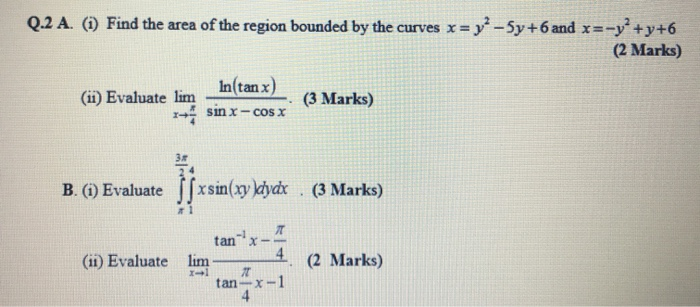(i) Find the area of the region bounded by the curves x = y 5y+6 and x =-y +y+6 Q.2 A. (1) Find the area of the region bounded by the curves x = y2 - 5y +6 and x=-y+y+6 (2 Marks) In(tan x) (ii) Evaluate lim (3 Marks) sinx-cosx B. (1) Evaluate |fxsin(xy dydx (3 Marks) X- (1) Evaluate lim * (11) Evaluate tan lim- (2 Marks) 2 Marks) - tan

• ### 4. Sketch the region enclosed by the curves y = x, y = 4x, y =...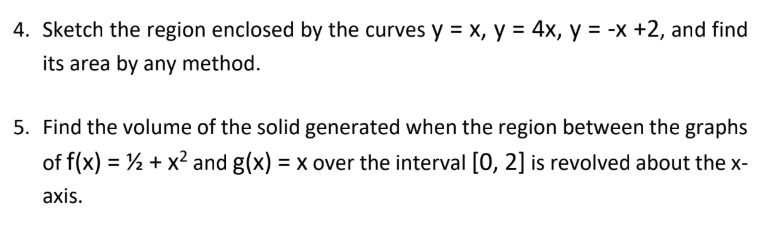4. Sketch the region enclosed by the curves y = x, y = 4x, y = -x +2, and find its area by any method. 5. Find the volume of the solid generated when the region between the graphs of f(x) = 1 + x2 and g(x) = x over the interval (0, 2) is revolved about the x- axis.

• ### 1. Find the mass and centroid of the region bounded by the = y2 with p...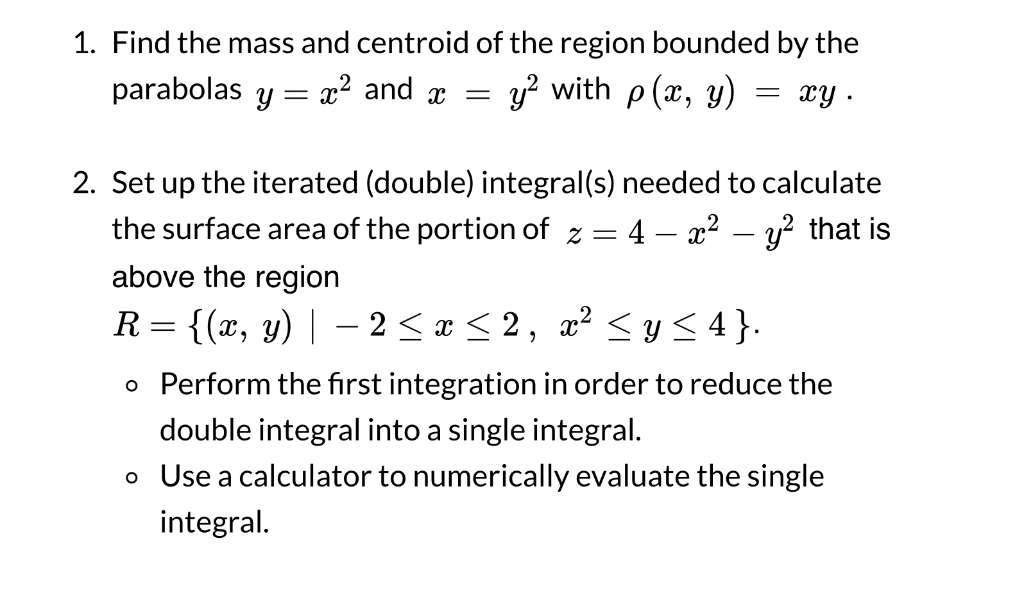1. Find the mass and centroid of the region bounded by the = y2 with p (a, y) parabolas y x2 and x 2. Set up the iterated (double) integral(s) needed to calculate the surface area of the portion of z 4 2 that is above the region {(«, у) | 2, x < y4} R 2 Perform the first integration in order to reduce the double integral into a single integral. Use a calculator to numerically evaluate the single...

• ### Find the area of the region enclosed by the following curves. (10 Puan) y = x2...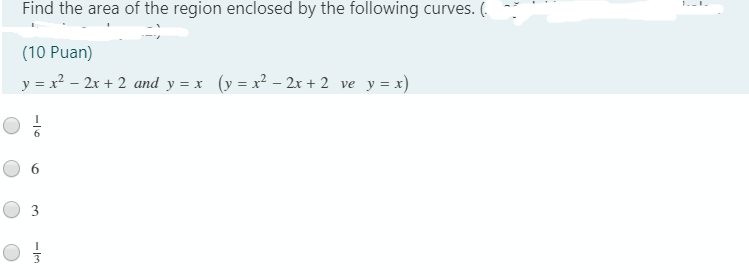Find the area of the region enclosed by the following curves. (10 Puan) y = x2 – 2x + 2 and y = x (y = x2 – 2x + 2 ve y = x) O 1 3